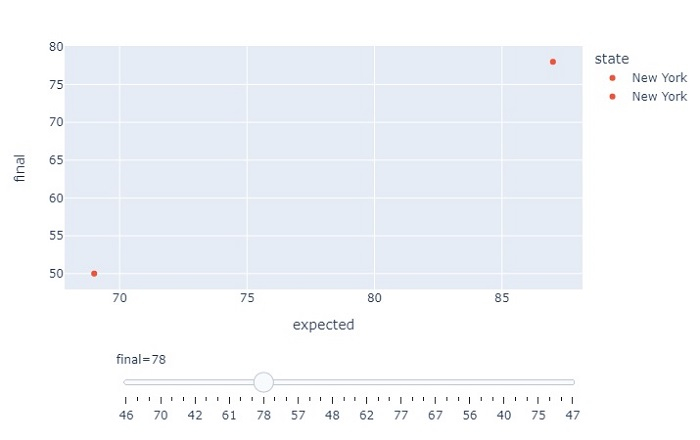# How to show a Plotly animated slider in Python?

Plotly supports different types of charts. In this tutorial, we will show how you can use Plotly to show an animated slider.

• We will use plotly.express used to generate figures. It contains a lot of methods to customize chart.

• To create a slide and set the frame, we will use the px.scatter() method and its attributes animation_frame and animation_group.

Follow the steps given below to show the animated slider.

Step 1

Import plotly.express module and alias as px.

import plotly.express as px


Step 2

Import the Pandas module and alias as pd.

import pandas as pd


Step 3

Import data from a CSV file using Pandas and create a dataframe.

import pandas as pd
df = pd.DataFrame(data)


Step 4

Create an animation frame and group using the following coordinates

fig = px.scatter(
df, x="expected", y="final",
animation_frame="final",
animation_group="state",
color="state", hover_name="state"
)


Step 5

Update the layout and display the figure using the following steps −

fig["layout"].pop("updatemenus")
fig.show()


## Example

The complete code to show animated slider is as follows −

import plotly.express as px
import pandas as pd

df = pd.DataFrame(data)
fig = px.scatter(df, x="expected", y="final", animation_frame="final", animation_group="state", color="state", hover_name="state")

# optional, drop animation buttons

fig.show()


## Output

On execution, it will show the following output on the browser −Observe that we got a slider at the bottom of the chart that you can operate which will in turn control the coordinates of the points on the chart.

Updated on: 21-Oct-2022

1K+ Views